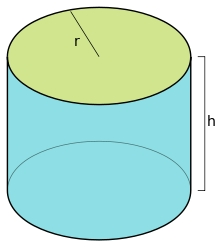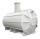# Cylinder height

Calculate the height of the cylinder and its surface is 2500 dm2 and the bases have a diameter 5dm.

h =  156.6549 dm

### Step-by-step explanation:Did you find an error or inaccuracy? Feel free to write us. Thank you!#### You need to know the following knowledge to solve this word math problem:

We encourage you to watch this tutorial video on this math problem:

## Related math problems and questions:

• Volume and surfaceCalculate the volume and surface area of the cylinder when the cylinder height and base diameter is in a ratio of 3:4 and the area of the cylinder jacket is 24 dm2.
• CylinderThe cylinder surface is 922 dm2. Its height is equal to the radius of the base. Calculate the height of this cylinder.
• Height as diameter of baseThe rotary cylinder has a height equal to the base diameter and a surface of 471 cm2. Calculate the volume of a cylinder.
• Diameter = heightThe cylinder's surface, the height of which is equal to the diameter of the base, is 4239 cm square. Calculate the cylinder volume.
• PrismCalculate the height of the prism having a surface area 448.88 dm² wherein the base is square with a side of 6.2 dm. What will be its volume in hectoliters?
• Equilateral cylinderEquilateral cylinder (height = base diameter; h = 2r) has a volume of V = 199 cm3 . Calculate the surface area of the cylinder.
• The cylinder baseThe cylinder with a base of 8 dm2 has a volume of 120 liters. From a cylinder filled with water, 40 liters of water was removed. At what height from the bottom /with precision to dm/ is the water level?
• 3rd dimensionThe block has a surface of 42 dm2 and its dimensions are 3 dm and 2 dm. What is the third dimension?
• Cylinder:Cylinder: calculate the height of a 3 liter cylindrical pot with a diameter of 10 cm.
• The cylinderThe cylinder has a surface area of 300 square meters, while the cylinder's height is 12 m. Calculate the volume of this cylinder.
• RollerCylinder shell has the same content as one of its bases. Cylinder height is 15 dm. What is the radius of the base of the cylinder?
• Cylinder - h2Cylinder volume is 2.6 liters. Base area is 1.3 dm2. Calculate the height of the cylinder.
• Perimeter of baseThe circumference of the base of the rotating cylinder is same as its height. What is the diameter and height of this cylinder with volume 1 liter?
• Surface of the cylinderCalculate the surface of the cylinder for which the shell area is Spl = 20 cm2 and the height v = 3.5 cm
• Edge of prismThe regular quadrilateral prism has a surface of 250 dm2, its shell has a content of 200 dm2. Calculate its leading edge.
• PotCalculate the height of 3 liter pot with shape cylinder with a diameter of 10 cm.
• SquareCalculate the area of the square shape of the isosceles triangle with the arms 50m and the base 60m. How many tiles are used to pave the square if the area of one tile is 25 dm2?